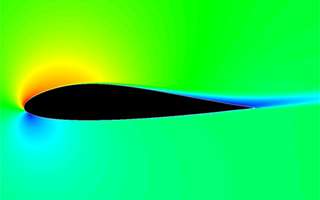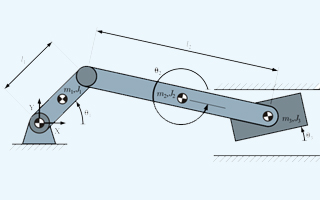##### Finite Element Analysis (FEA)In mathematics, finite element method (FEM) is a numerical technique for finding approximate solutions to boundary value problems. It uses variation methods (the calculus of variations) to minimize an error function and produce a stable solution. Analogous to the idea that connecting many tiny straight lines can approximate a larger circle, FEM encompasses all the methods for connecting many simple element equations over many small subdomains, named finite elements, to approximate a more complex equation over a larger domain.The finite element method (FEM) is the dominant discretization technique in structural mechanics. The basic concept in the physical interpretation of the FEM is the subdivision of the mathematical model into disjoint (non-overlapping) components of simple geometry called finite elements or elements for short. The response of each element is expressed in terms of a finite number of degrees of freedom characterized as the value of an unknown function, or functions, at a set of nodal points.

Finite Element Modelling under Static, Dynamic, Linear, Non-linear conditions covering

• Finite Element Meshing / Modeling
• Stress Analysis - linear/non-linear
• Thermal analysis
• Crash and Impact analysis
• Fatigue and durability analysis
• Harmonic analysis
• Noise & Vibration Analysis

Simgrosys supports to predict stress distribution, structural deformation and thermal deformation of mechanical and electronic components

##### Finite Element Analysis (FEA)In mathematics, finite element method (FEM) is a numerical technique for finding approximate solutions to boundary value problems. It uses variation methods (the calculus of variations) to minimize an error function and produce a stable solution. Analogous to the idea that connecting many tiny straight lines can approximate a larger circle, FEM encompasses all the methods for connecting many simple element equations over many small subdomains, named finite elements, to approximate a more complex equation over a larger domain.The finite element method (FEM) is the dominant discretization technique in structural mechanics. The basic concept in the physical interpretation of the FEM is the subdivision of the mathematical model into disjoint (non-overlapping) components of simple geometry called finite elements or elements for short. The response of each element is expressed in terms of a finite number of degrees of freedom characterized as the value of an unknown function, or functions, at a set of nodal points.

Finite Element Modelling under Static, Dynamic, Linear, Non-linear conditions covering

• Finite Element Meshing / Modeling
• Stress Analysis - linear/non-linear
• Thermal analysis
• Crash and Impact analysis
• Fatigue and durability analysis
• Harmonic analysis
• Noise & Vibration Analysis

Simgrosys supports to predict stress distribution, structural deformation and thermal deformation of mechanical and electronic components

##### Computational Fluid Dynamics (CFD)The aerodynamics deals with the flow of gases (especially air) over bodies such as aircraft, rockets, and automobiles at high or low speeds. Understanding motion of air around an object enables the calculation of forces and moments acting on the object. Typical properties calculated for a flow field include velocity, pressure, density and temperature as a function of spatial position and time. Aerodynamics allows the definition and solution of equations for the conservation of mass, momentum, and energy in air. Simgrosys supports aerodynamics design of automotive body shapes through mathematical analysis, empirical approximations, wind tunnel experimentation, and computer simulations.

Simgrosys consists of experts who can perform external aerodynamics study of flow around solid objects of various shapes. Evaluating the lift and drag on an airplane or the shock waves that form in front of the nose of a rocket are examples of external aerodynamics. Simgrosys also performs internal aerodynamics study of flow through passages in solid objects. For instance, internal aerodynamics encompasses the study of the airflow through a jet engine or through an air conditioning pipe. We solve the problems at subsonic, transonic, hypersonic at different speed conditions.

• CFD modeling / Meshing
• Internal and external flows
• Incompressible and compressible flows
• Multiphase flows
• Reactive flows

##### Multi body dynamics (MBD)Simgrosys supports to model the dynamic behavior of interconnected rigid or flexible bodies, each of which may undergo large translational and rotational displacements. The systematic treatment of the dynamic behavior of interconnected bodies has led to a large number of important multibody formalisms in the field of mechanics. Basically, the motion of bodies is described by their kinematic behavior. The dynamic behavior results from the equilibrium of applied forces and the rate of change of momentum. Nowadays, the term multibody system is related to a large number of engineering fields of research, especially in robotics and vehicle dynamics. As an important feature, multibody system formalisms usually offer an algorithmic, computer-aided way to model, analyze, simulate and optimize the arbitrary motion of possibly thousands of interconnected bodies.

A multibody dynamic (MBD) system is one that consists of solid bodies and/or links, that are connected to each other by joints that restrict their relative motion. The study of MBD is the analysis of how mechanism systems move under the influence of forces, also known as forward dynamics. A study of the inverse problem, i.e. what forces are necessary to make the mechanical system move in a specific manner is known as inverse dynamics.

Motion analysis is important because product design frequently requires an understanding of how multiple moving parts interact with each other and their environment. From automobiles and aircraft to washing machines and assembly lines - moving parts generate loads that are often difficult to predict. Complex mechanical assemblies present design challenges that require a dynamic system-level analysis to be met.

Accurate modeling can require representations of various types of components, like electronic controls systems and compliant parts and connections, as well as complicated physical phenomena like vibration, friction and noise. Motion analysis enables one to meet these challenges by quickly evaluating and improving designs for important characteristics like performance, safety and comfort.

• Rigid and flexible multibody systems
• Sensitivity analysis
• Vibration analysis
• Vehicle design & testing
• Coupled control/mechanical system analysis
• Kinematics and kinetics
• Contact and friction
• Loads and displacement
• Durability and life-cycle analysis
• Fracture or fatigue calculations
• Kinetic, static, and dissipative energy distribution
• Vehicular cornering, steering, quasi-static, and straight-line analysis
• Control system analysis
Simgrosys can support the following industries multibody applications:
• Automotive: Suspension systems, Drivetrains, brake systems, steering systems, engines, control systems, transmissions, boot joints, bearings, clutches, chassis structure.
• Manufacturing: robotic manipulators, conveyor belts, pumps, machine tools, packaging equipment, gears, stepper and server motors.
• Heavy Equipment: excavators, agricultural equipment, hydraulic control systems, tracked vehicles, fork lifts, amusement park rides.
• Medical: ergonomic analyses, robotic limbs.
• Consumer Products: Sporting goods, bicycles, tools, printers.
• Energy: Wind turbines, solar panels, offshore structures, drilling rigs, centralizer mechanisms.

##### Acoustics

We have excellent infrastructure capacity to measure the noise level and quality using techniques such as FFT and devise control techniques.

• Fluid borne noise
• Structural borne noise
• Aero acoustics